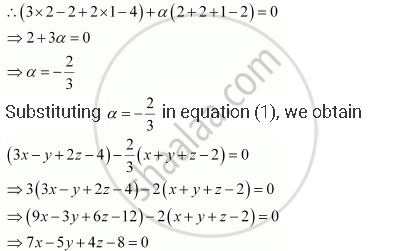Share

# Find the Equation of the Plane Through the Intersection of the Planes 3x – Y + 2z – 4 = 0 and X + Y + Z – 2 = 0 and the Point (2, 2, 1). - CBSE (Science) Class 12 - Mathematics

ConceptPlane Plane Passing Through the Intersection of Two Given Planes

#### Question

Find the equation of the plane through the intersection of the planes 3x – y + 2z – 4 = 0 and x + y + z – 2 = 0 and the point (2, 2, 1).

#### Solution

The equation of any plane through the intersection of the planes, 3x − y + 2z ­− 4 = 0 and x + y + z − 2 = 0, is

3x − y + 2z ­− 4 + alpha(x + y + z − 2) = 0 where alpha in R ....(1)

The plane passes through the point (2, 2, 1). Therefore, this point will satisfy equation (1).This is the required equation of the plane.

Is there an error in this question or solution?

#### APPEARS IN

NCERT Solution for Mathematics Textbook for Class 12 (2018 to Current)
Chapter 11: Three Dimensional Geometry
Q: 9 | Page no. 493
Solution Find the Equation of the Plane Through the Intersection of the Planes 3x – Y + 2z – 4 = 0 and X + Y + Z – 2 = 0 and the Point (2, 2, 1). Concept: Plane - Plane Passing Through the Intersection of Two Given Planes.
S